# Percentage - fractions

About what percentage we must increase number 1/6 to get number 1/3?

x =  100 %

### Step-by-step explanation:

(1+x/100)•1/6 = 1/3

0.5x = 50

x = 100

Our simple equation calculator calculates it.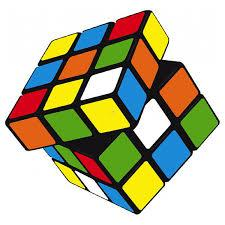Did you find an error or inaccuracy? Feel free to write us. Thank you!Tips to related online calculators
Do you have a linear equation or system of equations and looking for its solution? Or do you have a quadratic equation?

## Related math problems and questions:

• Percentage increaseIncrease number 400 by 3.5%
• Magnification of the square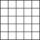If we increase the square side, increase the content of the 70 %. About what percentage was increased his sides?
• Percentage increaseWhat is the annual percentage increase in the city when the population has tripled in 20 years?
• Salary increase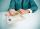Ms. Merry's salary increased by 15%, and that was 83 euros. What should pay before the increase?
• Price increase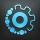A 20 percent price increase meant a 90-crown raise. How many cost a product after?
• Secret number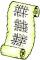Determine the secret number n, which reversed decrease by 16.4 if the number increase by 16.4.
• TVsProduction of television sets increased from 3,500 units to 4,200 units. Calculate the percentage of production increase.
• What percentageWhat percentage is 12 km out of 80 km?
• If weIf we increase the unknown number by 4%, we get 780. Determine the unknown number.
• Summer camp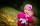84 children spent their holidays at the summer camp. There were 8 fewer boys than girls. What percentage were boys and what percentage were girls?
• Unknown number 10Number first increased by 30%, then by 1/5. What percentage we've increased the original number?After two hours and 40 minutes, the job is finished. Compared to last year, we were 40 minutes faster. What is the percentage increase to our performance?About what percentage increases perimeter and area of a rectangle if both the sides 12 cm and 10 cm long we increase by 20%?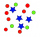The ratio of two number is 5:4 if 40% of the first number is 12, what will be 50% of the second number?The number 72 increase by 25%. By how much % will you have to reduce the number you created to get the number 72 again?I think of an unknown number. If we enlarge it five times, then subtract 3, and the result decreases by 75%, we get one greater than the number. What number am I thinking of?The numbers of students with honors in 2013 and 2014 are in ratio 40:49. How big is the year-on-year percentage increase?